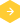# Will dbBee show calculated fields?

dbBee only reads data from data sources (Excel workbook or Access database), ignoring all the formatting, macros, charts, reports, forms, etc. However, calculated fields can be used as read-only fields (you can not add/update them using CD / Admin / CUD wizards) and in case of Excel sheet, they have to be calculated using the Excel program.

This means that in case of Access database, if you have a calculated field named "Amount" with expression Price*Quantity, it is enough to add values to fields Price and Quantity and field Amount will be automatically calculated. On the other hand, if you have same field in Excel sheet ("Amount" with formula "=C1*D1" where C1 and D1 are cells in columns Price and Quantity respectively), you can add values to fields Price and Quantity but your field Amount will NOT be calculated until you download your excel workbook and open it in Excel.
BACK
RELATED FAQCan I make charts using dbBee?What is the difference between the idbbee and edbbee plugins?Can you delete some of my projects? I did not realize I was creating more than one.Can I make a copy of my project?How do I rename my project?What happens when I exceed my monthly bandwidth quotaWhat is the project?